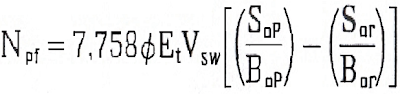# PREDICTION OF THE RECOVERY OF OIL BY WATER INJECTION

The calculations for prediction need not be very sophisticated to obtain reasonable accuracy of the volume to recover the parameters that are involved in the equations should be as accurate as possible and that a variation of 0.04 or 0.05% porosity reservoir level can reservoir thousand barrels were significant.

The general equation for volume of oil recovery by water injection (NPF) is:Where:

* Et: total efficiency
* VSW: Gross volume flood
* Sop: So (oil saturation) at the start of injection
* Bop: FVF (formation volume factor) at the start of injection
* Sister: So (oil saturation) the abandonment of the injection
* Bor: FVF (formation volume factor) the abandonment of the injection

* The 7.758 is due to the conversion of units, put another way: the formula is made to apply in barrels (volume) and acre-feet (area)

This equation is based on the difference between oil in the VSW to the start of injection and oil in the VSW to the abandonment of the injection. A common mistake is to assume that the flood volume is equal to the total volume of the reservoir without making the necessary adjustments.

Not all the oil included in the VSW is swept by the water, this is corrected by the Et.

It should consider each of the individual elements of the equation. Often VSW considerations eliminates the areas where there is marginal porosity.

In Bor assessment should be remembered that probably will not have much gas in solution than you had at the start of injection.

Free gas trapped gas can be returned to solution by the increased pressure due to the injection, but this amount is usually negligible.

Not commit a mistake when you consider that the Bop = Bor, so for practical purposes if no data are available separately, can be considered equal to Bop Bor.

Determination of oil saturation at the start of injection

Is determined by an equation of material balance, based on the total volume of the reservoir:It assumes a uniform saturation throughout the entire reservoir.

Where:

* Npp: cumulative oil production at the beginning of the injection (production history)
* N: original oil insitu
* Boi: Initial FVF
* Sw: Water saturation

The original oil insitu (N) can be calculated by BM (Material Balance) or volumetrically:

Where:

* CBD: Gross volume
* SWC: Water Saturation